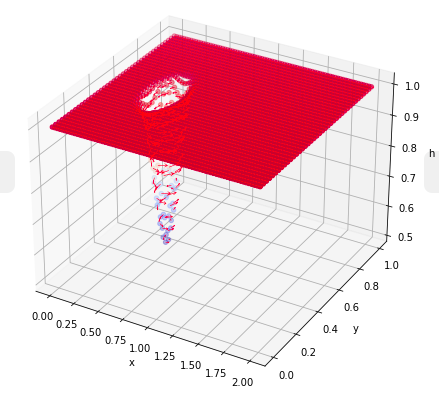# Analytical traveling vortex solutions of hyperbolic equations for validating very high order schemes

Published in arXiv, 2021

Recommended citation: M. Ricchiuto and D. Torlo. (2021). "Analytical traveling vortex solutions of hyperbolic equations for validating very high order schemes. " arXiv preprint, https://arxiv.org/abs/2109.10183. https://arxiv.org/abs/2109.10183

This is a work in collaboration with Mario Ricchiuto.

Testing the order of accuracy of (very) high order methods for shallow water (and Euler) equations is a delicate operation and the test cases are the crucial starting point of this operation. We provide a short derivation of vortex-like analytical solutions in 2 dimensions for the shallow water equations (and, hence, Euler equations) that can be used to test the order of accuracy of numerical methods.

• There are non compact vortexes, which are well known in literature, based on Gaussians shapes, hence suited for arbiratrily high order schemes. These are very simple to integrate and derivate, so their analytical form is particularly managable. On the other side one has to be careful with the boundaries. Choosing the domain large enough one can reach machine precision close at the boundary of the domain and obtain the goal.
• Another formulation used more in the Shallow water community is the one proposed in Ricchiuto, M., & Bollermann, A. (2009). Stabilized residual distribution for shallow water simulations. Journal of Computational Physics, 228(4), 1071-1115. It is based on a cosine function restricted on an disk. This solution, though being compact supported, is not smooth. Indeed, the solution for water height has continuous derivative up to the 4th one, while the discharge only up to the 2nd. So the test is not suited to study the order of accuracy of methods with more than second order. We introduce a generalization of such solutions with 2p continuous derivatives in the discharge, which can be used with any method.
• A final class of vortexes is presented where the integral analytical formula used from the previous vortexes is exploited in an derivative sense. Hence, the complicated integral computations can be substituted by a simple derivation. It is then easy to start from a smooth compact supported bump for the perturbation of the water height and to derive the analytical formula for the discharge.